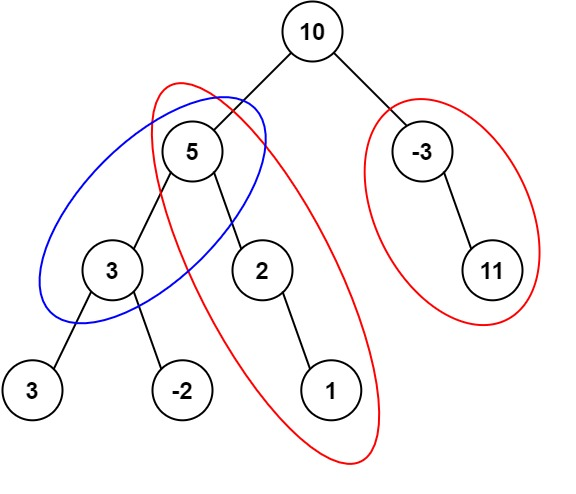# 437. Path Sum III

## 437. Path Sum III

Given the `root` of a binary tree and an integer `targetSum`, return the number of paths where the sum of the values along the path equals `targetSum`.

The path does not need to start or end at the root or a leaf, but it must go downwards (i.e., traveling only from parent nodes to child nodes).

Example 1:```Input: root = [10,5,-3,3,2,null,11,3,-2,null,1], targetSum = 8
Output: 3
Explanation: The paths that sum to 8 are shown.
```

Example 2:

```Input: root = [5,4,8,11,null,13,4,7,2,null,null,5,1], targetSum = 22
Output: 3
```

Constraints:

• The number of nodes in the tree is in the range `[0, 1000]`.
• `-109 <= Node.val <= 109`
• `-1000 <= targetSum <= 1000`

### The solution to the Above Question

/**
* Definition for a binary tree node.
* struct TreeNode {
*     int val;
*     TreeNode *left;
*     TreeNode *right;
*     TreeNode() : val(0), left(nullptr), right(nullptr) {}
*     TreeNode(int x) : val(x), left(nullptr), right(nullptr) {}
*     TreeNode(int x, TreeNode *left, TreeNode *right) : val(x), left(left), right(right) {}
* };
*/
class Solution {
public:
int count=0;

int ans=0;
void helper(TreeNode* rootint target,int sum){
if(root==NULL){
return;
}
int data=root->val;
if(data==(target-sum)){
count++;
}
sum+=data;
helper(root->left,target,sum);
helper(root->right,target,sum);

}

int pathSum(TreeNode* rootint targetSum) {
helper(root,targetSum,0);
if(root==NULL){
return 0;
}
ans+=pathSum(root->left,targetSum);
ans+=pathSum(root->right,targetSum);
return count;
}
};